Courses

# Potential And Current Transformers Electrical Engineering (EE) Notes | EduRev

## Electrical Engineering (EE) : Potential And Current Transformers Electrical Engineering (EE) Notes | EduRev

The document Potential And Current Transformers Electrical Engineering (EE) Notes | EduRev is a part of the Electrical Engineering (EE) Course Electrical Machines.
All you need of Electrical Engineering (EE) at this link: Electrical Engineering (EE)

Potential and Current Transformers
(Refer Slide Time: 00:37)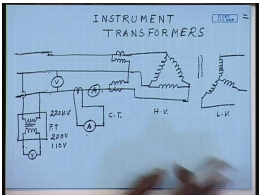So far we have seen transformers being used for stepping up or stepping down voltages for supplying power to different types of loads. For example, a three phase transformer can be connected like this for stepping up or stepping down the voltage to supply a load. This is let us say from high voltage side to low voltage side and vice versa. Now in many cases it becomes necessary to measure the line voltage or the current flowing through the line. Ordinarily one would expect to put a voltmeter and may be in series an ammeter and if you have to measure power, then possibly two wattmeters.

For two wattmeter method of power measurement, it should be connected; however, in power transmission this line voltage can be in excess of 200 kilovolt. This current will be in excess of several kilo amperes. So, instruments which can measure directly that kind of voltage or current are not available. Even if there made available, working them with at such high voltages and currents is not desirable from the safety point of view. Hence, it is necessary to reduce this voltage and current magnitudes in order to bring them to the level where they can be safely measured using again transformers. These are called instrument transformers.

There are two types. One is used to reduce the voltage level and hence called the potential transformer or the P.T. This is the P.T to the secondary of which we can connect the voltmeter. It steps down the voltage let us say from some 200 kilovolt. So, may be to 220 volts or more often than not 110 volts. And a current transformer that steps down the current to which ammeter can be connected to a much lower value of the ratio of 100 or even more. So, this is called the current transformer or in short C.T. Now the working principle of these transformers are similar to normal single phase transformers; however, special care has to be taken, so that the voltage appearing across the voltmeter here or the current flowing through the ammeter here are in proportional proportion to and in phase with the high voltage side or high current side voltages and currents.

Normally these transformers will be loaded with the measuring coils of the instrument; the potential transformer will be connected to a voltmeter whereas the current transformer will be connected to an ammeter. So, a potential transformer will work almost at no load or at very light load. Similarly, the current transformer secondary will always be almost short circuited. So, let us see what type of precaution or what type of constructional specialties is needed to make this transformer. Let us take up the case of a potential transformer first.

(Refer Slide Time: 06:32)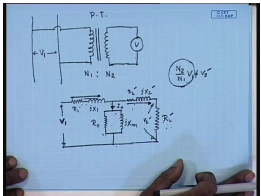As the name suggests the potential transformer is used to step down a high primary side voltage to a lower voltage of the range of 110 volts and is loaded usually with a volt current pressure voltmeter or the pressure coil of a wattmeter; both of which has very large impedance. So, let us say the turns ratio of this is N 1 to N 2. The equivalent circuit of this P.T refer to the high voltage side will be something like magnetization branch. This is R 1, this is j X 1, this is R 0 j X N. This is the primary side line voltage V 1; this is R 2 dash; this is j X 2 dash, and this is the R L dash which is the resistance of the voltmeter.

Obviously, it should be noted that the voltmeter resistance is very large. The resistance of the voltmeter is very large, and hence the reflected resistance of the voltmeter can be comparable to the magnetization branch. Hence, it is necessary that the magnetization branch impedance of a potential transformer be very large. So, special care should be taken to make sure that the magnetization current of a P.T is very small. Even then when the magnetization impedance is small it cannot be met lower than a limit; there will be some drop in the series impedances.

Hence, we know that the output voltage presented to the load will differ from the value N 2 by N 1 into V 1 V 2 dashed under the applied load will not usually be N 2 by N 1 into V 1; both the magnitude and the phase angle of V 2 dashed will be different from this quantity. This is the ideal voltage that the voltmeter should have measured. However, due to the presence of the magnetization current as well as the series impedances, the actual voltage presented to the voltmeter will be different from its nominal value and the actual voltage will in fact depend on the voltmeter or the load connected to the P.T.

Therefore, few things are in order. First of all, the magnetizing impedance of the P.T should be very small; second, its leakage impedances should also be very small so that the regulation of the P.T is almost zero. Third, it is desirable that a P.T we matched with a measuring instrument; that is if a P.T needs to be calibrated when you connect a voltmeter, you must calibrate the actual voltage and the voltage measured. So, it is necessary that a P.T is used with a particular voltmeter so that the current drawn by the meter does not change.

Even then it will change to some extent as V 1 changes but at least it can reduce this; the regulation will be negligible. Hence, P.T series impedance will be very close to zero. It is imperative that the secondary of the P.T should never even accidentally be short circuited because then a very large current will flow; its impedances are almost zero and will destroy the instrument. So, let us see what will be the relationship between the voltage V 2 dash and V 1.

(Refer Slide Time: 13:01)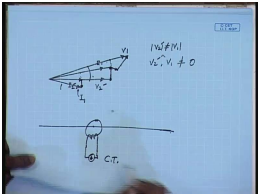This is the P.T equivalent circuit is somewhat like this; let us say this is V 2 dash. A voltmeter will almost appear as a resistive load. So, this is I 2 dash. Hence, the no load voltage E 1 will be V 2 dashed plus I 2 dashed R 2 dash. This would be the no load voltage V 1 or E 1. The no load current will lag E 1 by almost 90 degree and should be very small compared to load current. So, this will be the current I 1 drawn by the P.T primary, and the voltage V 1 will be E 1 plus I 1 R 1 plus j X 1. This is the angle; this is the voltage V 1.

So, we see that because of the impedance drop V 2 dash is not equal to V 1; mod of V 2 dashed is not equal to V 1 and the angle between V 2 dashed and V 1 is also not zero. Hence, in voltage measurement there will be an error introduced due to the series impedance and when power measurement not only the magnitude but the phase angle error between V 1 and V 2 dashed will become important. So, constructional features should address these problems.

So, one should make sure that the no load current is very small as well as the series impedances of the potential transformer are very small. For the current transformer, what normally is done is that the current carrying conductor itself, but the line itself acts as a primary. This normally passes through a magnetic core the current carrying primary and the secondary are on the magnetic core to which an ammeter is connected. So, if we draw the equivalent circuit of a C.T.

(Refer Slide Time: 17:06)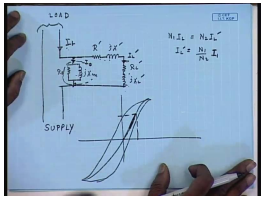For a current transformer it will be somewhat like, the primary of the C.T is in series with the line. Hence, this is the primary of the C.T. This is the magnetization branch of the C.T referred to the primary side. This is connected to the load; this is supply. This is R 0; this is j X M; this is R dash j x dash; this is R L dash; this is j X L dash. Few things to note; first of all the load current I L flowing here and I L dashed flowing here ideally should be related by N 1 I L equal to N 2 I L dash, or I L dash should be equal to N 1 by N 2 into I L.

However, that does not happen, because the magnetization branch of the C.T shunts a part of the current I 0. Hence, again it is important that the magnetization branch impedance of the C.T be negligible compared to the rest of the impedance that is the series impedance plus the load impedance. So, it is necessary that C.T magnetization impedance be kept low; however, there is another important point here. It can be seen that even by accident if the load is open circuited, then relatively large magnetization branch impedance is put in series with the line but even then that impedance is small compare to the load.

Hence, this impedance does not affect the load current much, but what it does is the voltage across the C.T voltage drop across the C.T increases very much, and along with that the flux density in the C.T and the C.T coat saturate. Once it saturates its properties changes and hence that C.T cannot be used any longer. So, care should be taken that a C.T is not C.T secondary is one; the current is flowing through the primary, the secondary of the C.T should not be open circuited even accidentally. Another important point for C.T is that unlike a potential transformer in a potential transformer the applied voltage to the primary remains more or less constant varying within nominal range.

Hence, this transformer can be designed in the manner similar to normal transformers or power application; however, for a C.T the voltage applied across the primary of the C.T depends on the multiple products of the load current and the meter instrument impedance. If we use the same meter, then this impedance will remain constant; even then the voltage applied across a C.T is proportional to the current. Hence, a C.T always operates at a variable applied voltage and hence at variable flux density. Therefore, in order to have a linear or a fixed relationship, it is necessary that the C.T operates in the linear region of the b H characteristics of the material, so that the impedances does not depend exactly on the applied voltage and a linear circuit model can be used for a C.T.

(Refer Slide Time: 22:56)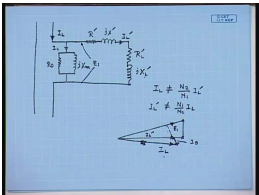If we try to draw the phasor diagram of a C.T, this is the series impedance of the C.T itself, and this is the impedance of the instrument may be an ammeter or the current coil of a wattmeter. This current is I L dash. To draw the phasor diagram let us say this is I L dash. So, the voltage E 1 will be I L dash into the total impedance I L dashed into R L dash plus R r dash plus I L dashed into X L dashed j I L dashed X L dash plus X L dash. So, this is the potential E 1. The no load current will be almost at 90 degree with E 1. This is I 0. Hence, this will be the current I L. Again we see that the current I L is not equal to N 2 by N 1 I L dash, or I L dash is not equal to N 1 by N 2 into I L.

It will depend on the relative magnitude of I L and I 0. In order for this current to be this calibration to hold, it is necessary also to make the C.T magnetization branch impedance of the C.T magnetization branch as high as possible. Otherwise, it will introduce both magnitude error and the phase error. For current measurement the magnitude error only is important. But when you want to measure power delivered to a load using potential and current transformer in addition to the magnitude error, we should also take care of the phase error introduced by both this transformers; we will see this with the help of an example.

(Refer Slide Time: 28:01)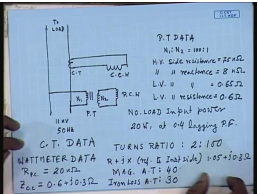Let us say I have an 11 kV source 11 kV 50 hertz source, and it is applying power to a load. I want to measure this power using a wattmeter and a potential transformer using a C.T, P.T and potential and a wattmeter. So, what I will do? I will have a P.T connected here as well as I will have a C.T connected here. The wattmeter current coil will be, this is the C.T; this is the P.T wattmeter current coil. This is the current coil of the wattmeter, and the potential coil of the wattmeter will be connected across the P.T of the P.T secondary.

So, the data is something like this. The P.T data the turns ratio that is N 1 is to N 2, this is equal to 100 is to 1. This is the nominal conversion ratio also. So, H.V side resistance is 7.5 kilo ohm. H.V side reactance equal to 8 kilo ohm, L.V side reactance equal to 0.65 ohm, L.V side resistance equal to 0.6 ohm and no load input power of the P.T is 20 watt at 0.4 lagging P.F with rated voltage on the H.V side. The C.T data are similar turns ratio is 2 is to 200. The series impedance that is R plus j x this is referred to instrument side.

This is 1.05 plus j 0.3 ohm, magnetizing ampere turns is 40, iron loss ampere turns is 30. The wattmeter data resistance of the potential coil is 20 kilo ohm, whereas the impedance of the current coil is this. Now let us say the source is supplying 500 ampere at 0.9 lagging P.F and at rated voltage that is at 11 kV. Question is what will be the reading of this wattmeter? How much power will the wattmeter read? Let us try to find out.

(Refer Slide Time: 35:04)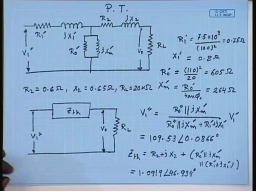Now for the P T as we have seen the equivalent circuit looks somewhat like this; that is the equivalent circuit of a normal transformer. This is for the P.T. This is R 1 dash, the voltage of light is V 1 dash j X 1 dash. This is R 2; this is j X 2; this is R L; this is the voltage V 0 that we decide by the potential coil of the; that will be read by the potential coil of the wattmeter. Now what is R 1 dash? From the given data R 1 dashed comes to 7.5 kilowatt divided by turns ratio square referred to the meter side. This is 0.75 ohm. Similarly, x 1 dash comes to 0.8 ohm. R 0 dash refer to the meter side will come to it is mentioned that it is 20 watt at when rated voltage was applied to the primary side; hence, the secondary voltage was also rated.

So, refer to the secondary side R 0 dash is 110 square by 20; that gives you 605 ohm, and X dash equal to R 0 dash by tan phi 0. This comes to 264 ohm. The resistance R 2 equal to 0.6 ohm. X 2 equal to 0.65 ohm and R L equal to 20 kilo ohm. Now we can draw the Thevinin equivalent circuit of the P.T equivalent circuit across the meter terminal. This will look somewhat like Thevinin impedance Z t h V 1 double dash R L, and this is V 0. Obviously, V 1 double dash the Thevinin voltage will be same as the voltage coming here divided by R 0 double dashed j X m dash plus R1 dash plus j X 1 dashed into V 1 dash.

This comes to when we substitute the values V 1 and taking V 1 dash to be 110, it comes to 109.53 angle of 0.0866 degrees and z Thevinin, which is given by R 2 plus j X 2 plus R 0 dashed parallel j X m dash parallel R 1 plus j R 1 dash plus j X 1 dash. This comes to 1.0919 angle 46.934 degrees.

(Refer Slide Time: 41:37)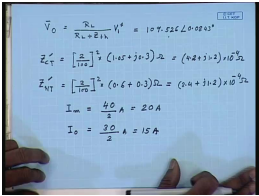Hence, the voltage V 0 will be R L divided by R L plus z Thevinin into V L double dash, and this comes to 109.526 angle 0.0843 degree. This is the voltage that will be applied across the pressure coil of the wattmeter instead of actual 110 angle 0; this is the voltage that will be applied. So, both there is a magnitude and a phase error. Now for the C.T, what is the C.T impedance referred to the supply side? That is Z C T dash equal to 2 by 100 which is the turns ratio square into the impedance is given referred to the meter side 1.05 plus j 0.3 ohm.

This comes to 4.2 plus j 1.2 into 10 to the power minus 4 ohm. Similarly, the impedance of the wattmeter current coil referred to the supply side will be 2 by 100 square into 0.6 plus 0.3 ohm. This will come to 2.4 plus j 1.2 into 10 to the power minus 4 ohm. The magnetization current referred to the supply side to the magnetization ampere turns divide by turns on the supply side by 2. This will be equal to 20 amperes and the iron loss is 30 magnetization amperes turns by 2; that is equal to 15 amperes.

(Refer Slide Time: 45:15)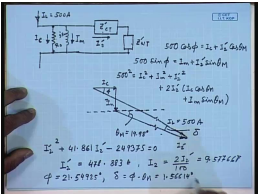So, the equivalent circuit on the C.T looks somewhat like this. This current the total current flowing is I L equal to 500 amperes. This current is I C. This current is I M; the current flowing to the C T is to the wattmeter pressure coil current coil is I 2 dash. Now we can draw the phasor diagram. We take I C to be this current, and this is the current I M there at right angles. And then the voltage across this combination is in phase with I C into R 0 and hence with I C. So, the voltage across this branch is in this direction. Hence, we can draw the current drawn by this circuit; the angle of that current will be the angle of the sum of this impedances. So, let us say this will be the angle.

This will be the current I 2 dash; the magnitude we still do not know, but we know this angle. This angle is theta M comes to 19.98 degree. This is the angle of the sum of these impedances; however, the total current I L should be the sum of I C plus I N plus I 2 dashed. This will be I L; you know this magnitude is 500 ampere. So, let us say this angle is delta, and this angle is phi. Now from this phasor diagram we can write that 500, this is the magnitude 500 cos phi equal to I C plus I 2 dash cos theta M and also 500 sin phi equal to I M plus I 2 dashed sin theta M.

Therefore, 500 square equal to I C square plus I M square plus I 2 dash square plus 2 I 2 dash I C cos theta M plus I M sin theta M, or from this equation we can simplify it to read I 2 dash square plus; since, all other values for example, theta M I C and I M are known 41.861 I 2 dash minus 249375 equal to 0. This gives the meter current referred to the supply side I 2 dash to be equal to 478.883 amperes. Hence, the actual current I 2 flowing through the meter is 2 I 2 dash by 100; that comes to 9.57766 ampere. The angle phi comes to be 21.54925 degrees and the angle delta which is phi minus theta M. This comes to1.56614 degrees.

(Refer Slide Time: 52:48)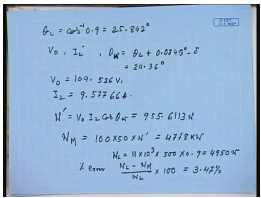The angle between supply voltage and supply current is theta L which is cos inverse 0.9 which is 25.842 degrees. The angle between V 0 that is the voltage across the pressure coil of the wattmeter and I 2 is theta W which is theta L plus the voltages angle is 0.0843 degrees minus delta. This comes to equal to 24.36 degrees, whereas magnitude of V 0 is 109.526 volts, and I 2 is equal to 9.57766 amperes. So, the power read by the wattmeter is V 0 I 2 cos of theta W; this comes to 955.6113 watts.

So, multiplying by the nominal C.T and P.T ratio, the wattmeter reading will be 100 into 50 into W dash; that is equal to 4778 kilowatt, whereas the actual power is 11 into 10 to the power 3 into 500 into 0.9 W load. This comes to 4950 watt. So, the percentage error due to this W L minus W M by W L into 100; this comes to 3.47 percent which is due to both angle error and the magnitude error of the C.T and the P.T respectively.

Thank you.

Offer running on EduRev: Apply code STAYHOME200 to get INR 200 off on our premium plan EduRev Infinity!

61 docs|20 tests

,

,

,

,

,

,

,

,

,

,

,

,

,

,

,

,

,

,

,

,

,

;